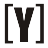# Amps (A) to volt-amps (VA) Calculator

To calculate Amps to VA, select phase (single or three phase) first, insert value of amps and line to line volts then press Calculate Button to get the result of volt-amps (VA). Swap to VA to Amps calculator.

 Select Phase #: Single phase Three phase Enter amps: A Enter line to line volts: V Result in volt-amps: VA

## How to Calculate Amps (A) to volt-amps (VA)

Single phase amps to VA Formula
`S (VA) = I (A) × V (V)`

Power S in volt-amps equal to current I in amps, multiply the voltage V in volts.

Three phase amps to VA Formula
Line to line voltage

`S (VA) = √3 × I (A) × VL-L (V)  = 3 × I (A) × VL-N (V)`

Power S in volt-amps equal to square root if 3 current I in amps, times the line to line voltage VL-L in volts.YoosFuhl.com Would you like to receive notifications on latest updates? No Yes